# Robust Neural Control of the Dual Star Induction Generator Used in a Grid-Connected Wind Energy Conversion System

Robust Neural Control of the Dual Star Induction Generator Used in a Grid-Connected Wind Energy Conversion System

Hamza Mesai-ahmedAbderrahim Bentaallah Antonio J. Marques Cardoso Youcef Djeriri Imed Jlassi

ICEPS Laboratory, Djillali Liabes University, PO Box 89, Sidi Bel-Abbes 22000, Algeria

CISE - Electromechatronic Systems Research Centre, University of Beira Interior, Covilha 6200-001, Portugal

Corresponding Author Email:
Hamzamesai2@gmail.com
Page:
323-332
|
DOI:
https://doi.org/10.18280/mmep.080301
4 June 2020
|
Accepted:
3 June 2021
|
Published:
24 June 2021
| Citation

OPEN ACCESS

Abstract:

This paper presents a field-oriented control (FOC) of a dual star induction generator (DSIG) applied in a grid-connected wind energy conversion system. Currently, the dual star induction machine (DSIM) is increasingly used among multiphase machines. The machine has two star-connections, sharing the same stator offset, by an electrical angle of 30° and fed by two parallel converters. Maximum power point tracking (MPPT) is illustrated in a first stage, in order to extract a maximum of power under fluctuating wind speed. In a second stage, vector control of a DSIG with FOC is described. Finally, voltage oriented control (VOC) is used to ensure the power factor unity on the grid side. The main contribution of the presented paper is the application of a simple architecture of an artificial neural network (ANN) controller in order to improve the robustness and stability of the system, especially against the parameter change. In comparison with the conventional control, which is known by its sensitivity, the proposed neural MPPT with neural FOC (NMPPT-NFOC) presents better performance under normal and abnormal conditions. The robustness and effectiveness of the proposed control has been validated through illustrative simulation results with different functional zones, and for fixed and variable wind speed.

Keywords:

wind energy, dual star induction generator (DSIG), ANN controller, PI controller

1. Introduction

Due to the environmental and global climate changes, renewable energy sources play a vital role in the energy market. Among several sources of renewable energy, wind energy is one of the most important and the most promising renewable source of energy throughout the world in terms of development, because it is non-polluting and economically viable . Wind energy is carried by the wind. With this energy, wind turbines can produce electrical energy .

The latest generation turbines operate at variable speed. This type of operation has many advantages, such as, operation at maximum power point tracking (MPPT) and higher energy efficiency with low voltage peaks on the components . Compared to fixed speed wind turbines, variable speed wind turbines have the advantage to be supervised by control algorithms, allowing the control of active and reactive powers injected into the grid .

Regarding the generators for wind energy conversion system (WECS), wind turbine manufacturers use several types of electric generators, notably doubly fed induction generators [5-7], permanent magnet synchronous generators [8, 9], and the dual star induction generator (DSIG) [10, 11]. The DSIG has more advantages than the conventional cage induction generator, namely high reliability, low magnitude with high frequency of torque pulsations, reduced rotor harmonic current, and segmentation power .

Actually, the main goal of researchers is to make efforts for improving the conventional control algorithms, which are known by their limitations according to the disturbances and parameter variation effects. For that reason, many techniques have been developed recently to control the DSIG, such as nonlinear back stepping control , and nonlinear sliding mode control used with Field Oriented Control (FOC) . The control of the produced power by the DC bus voltage regulation has been presented .

The most recent and integrated techniques in high power systems are artificial intelligence techniques, known for their great potential to be able to solve problems related to industrial processes, including the control, estimation and identification of the parameters of the variant systems . The goal of Artificial Intelligence (AI) is to design systems that can reproduce the behavior of humans in their reasoning activities . However, the main drawback of these techniques is the requirement of high-speed microcontrollers, especially when using complex intelligent controllers as suggested by Chekkal et al. , and Ameur & Kouzi . An optimized PI and fuzzy speed controllers for DSIG are presented, which give relatively good results; but this control is designed by two different intelligent techniques . The control of the DSIG drive using intelligent fuzzy logic control is presented, where the proposed control is not tested for different conditions . In the same time, the last-mentioned control uses a high number of fuzzy rules, which make it difficult to implement into the microcontroller, and becomes an expansive solution. The application of the neural network control for wind turbine is proposed by Yaichi et al. , which provides good results, but the proposed algorithm was based on a complicated neural controller.

Avoiding the aforementioned problems related to the sensitivity of PI controllers as well as the complexity of the intelligent controllers, an artificial neural network controllers based on a simple architecture is proposed in this paper, which is trained offline by a standard back-propagation algorithm using the input-output (error-target) history information of each PI controllers. The proposed ANN controllers do not require an accurate mathematical model of the considered system, which makes them independent of the system model, so that they may be a good solution to solve the problem of sensitivity against the machine parametric variation. The proposed controllers are applied only in the machine side converters in order to ensure robust performance of the DSIG in different operating conditions. Regarding the grid-side converter, the VOC based on the PI controllers is considered.

This work is presented as follows: the modeling of the wind generator and the MPPT are presented in section 3, and the DSIG model is provided in section 4. In section 5 the FOC-DSIG is presented. Next, the proposed ANN controller is given in section 6. In section 7 the VOC based on the PI controllers is analyzed, and the results are presented and discussed in section 8. Finally, some conclusions are given in section 8.

2. Power Generation System Description

To connect the wind turbines to the grid, various ac/dc/ac configurations have been developed by the wind turbine manufacturers. In this study the variable speed wind turbine system consists of a squirrel cage DSIG, which is driven by the wind turbine trough a gearbox. The control performance of the DSIG is ensured by the proposed control algorithm (NFOC-NMPPT) through converters 1 and 2. The converter 3 is driven by the VOC strategy, to keep the control of the dc-bus voltage and to control the active and reactive powers delivered to the grid. The overall system scheme is illustrated in Figure 1.

## 1.png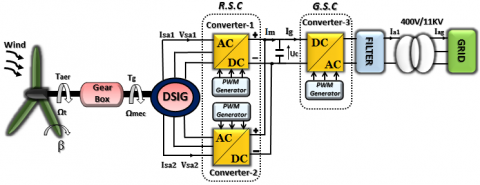Figure 1. Scheme of the studied system

3. Wind Turbine Model

The available power at a surface S, swept by the wind turbine is expressed as follows :

$P_{v}=\frac{1}{2} \rho \pi R^{2} V^{3}$  (1)

where, V is the wind speed (m/s), ρ is the density of the air, and R is the length of a blade or the radius of the wind turbine (m).

The wind turbine can recover only a fraction of the available power appears to the rotor, thus, the mechanical power of the turbine (Pt) can be represented by :

$P_{t}=C_{p}(\lambda, \beta) P_{v}=\frac{1}{2} \rho \pi R^{2} V^{3} C_{p}(\lambda, \beta)$  (2)

where, Cp (λ,β) is the power factor that characterizes the aerodynamic efficiency of the turbine (Figure 2). It depends on the dimensions of the blade, the angle of orientation of the blade β, and the ratio of the speed λ, and expressed as :

$C_{p}(\lambda, \beta)=$$0.5176\left(\frac{116}{\lambda_{i}}-0.4 \beta-5\right) \exp \left(\frac{-21}{\lambda_{i}}\right)+0.0068 \lambda$  (3)

where,

$\frac{1}{\lambda_{i}}=\frac{1}{\lambda+0.08 \beta}-\frac{0.035}{\beta^{3}+1}$  (4)

## 2.jpg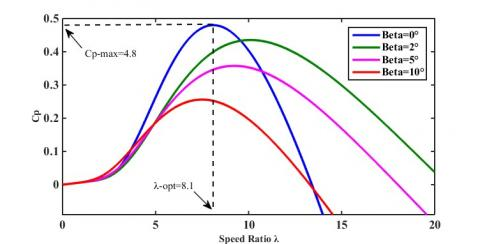Figure 2. Coefficient of the pair Cp, as a function of λ, for different β

The relative velocity λ is defined as the ratio between the linear speed of the blades (Ωt R) and the wind speed as follows [22-24]:

$\lambda=\frac{\Omega_{t} R}{V}$  (5)

where, Ωt (rad/s) is the mechanical speed of the turbine shaft.

The torque exerted by the wind on the turbine shaft is defined by:

$C_{t}=\frac{P_{t}}{\Omega_{t}}=\frac{1}{2} \rho \pi R^{3} V^{2} C_{c}(\lambda, \beta)$  (6)

where, Cc represents the torque coefficient:

$C_{c}=\frac{C_{P}}{\lambda}$  (7)

The gearbox conversion ratio (G), is designed to match the low-speed turbine blades with the high-speed generator. For a given rated speed of the generator and turbine, the gearbox ratio can be determined by :

$\Omega_{t}=\frac{\Omega_{g}}{G}$  (8)

$C_{g}=\frac{C_{t}}{G}$  (9)

where, t and g are the turbine and generator rated speeds.

According to the expressions (2) and (3), the maximal power generation of the wind turbine corresponds to the maximum value of the power coefficient. This is verified when the speed ratio was in its optimum value for β=0, as shown in Figure 2.

4. Dual Star Induction Generator Model

## 3.pngFigure 3. Representation model of the DSIG with stator-rotor orientation

The DSIG is a six-dimensional system, that is composed of two identical three-phase stator windings, and one squirrel cage rotor [26, 27]. The two split stator windings sets are connected in a star configuration, and are shifted by an electric angle a = 30° [10, 11]. The stator windings are being supplied by two independent converters. Because it is a six-dimensional system, the modeling of the DSIG using actual variables becomes a complicated task, and so the machine in this paper is being modeled in d–q reference frame, and expressed in state-space form. Certain assumptions have been taken into account in order to simplify the machine modeling [28, 29]:

• The stator windings are sinusoidally distributed.
• There is a uniform air gap between stators and rotor.
• Magnetic saturation of core is neglected.
• The two-stator windings are perfectly decoupled.

Figure 3 presents a model representation of the stator-rotor windings distribution for the DSIG. In this figure, (Sa1,Sb1,Sc1) and (Sa2,Sb2,Sc2) are the corresponding three-phase windings of the star1 and star 2, respectively. (ra,rb,rc) denote the rotor windings, and θs, θs-α are the angle position of the star 1 and star 2, respectively. The axes of each star's windings are displaced at an electric angle of 120°.

The mathematical model of the DSIG in state space form is presented by the following expressions [10, 18]:

$\left[ \overset{\bullet }{\mathop{I}}\, \right]=\left[ {{L}^{-1}} \right]\left\{ \left[ B \right]\left[ U \right]-{{\omega }_{gl}}\left[ C \right]\left[ I \right]-\left[ D \right]\left[ I \right] \right\}$  (10)

where,

$\omega_{g l}=\omega_{S}-\omega_{r}$

$\omega_{r}=p^{*} \Omega_{m e c}$

$[U]=\left[\begin{array}{llllll}V_{d s 1} & V_{q s 1} & V_{d s 2} & V_{q s 1} & V_{d r} & V_{q r}\end{array}\right]^{T}$

$[I]=\left[\begin{array}{llllll}I_{d s 1} & I_{q s 1} & I_{d s 2} & I_{q s 1} & I_{d r} & I_{q r}\end{array}\right]^{T}$

And:

$[B]=\operatorname{diag}\left[\begin{array}{llllll}1 & 1 & 1 & 1 & 0 & 0\end{array}\right]$

$\left[ C \right]=\left[ \begin{matrix} 0 & 0 & 0 & 0 & 0 & 0 \\ 0 & 0 & 0 & 0 & 0 & 0 \\ 0 & 0 & 0 & 0 & 0 & 0 \\ 0 & 0 & 0 & 0 & 0 & 0 \\ 0 & -Lm & 0 & -Lm & 0 & -\left( Lr+Lm \right) \\ Lm & 0 & Lm & 0 & Lr+Lm & 0 \\\end{matrix} \right]$

$\left[ L \right]=\left[ \begin{matrix} \left( Ls1+Lm \right) & 0 & Lm & 0 & Lm & 0 \\ 0 & \left( Ls1+Lm \right) & 0 & Lm & 0 & Lm \\ Lm & 0 & \left( Ls2+Lm \right) & 0 & Lm & 0 \\ 0 & Lm & 0 & \left( Ls2+Lm \right) & 0 & Lm \\ Lm & 0 & Lm & 0 & \left( Lr+Lm \right) & 0 \\ 0 & Lm & 0 & Lm & 0 & \left( Lr+Lm \right) \\\end{matrix} \right]$

$\left[ D \right]=\left[ \begin{matrix} Rs1 & -ws\left( Ls1+Lm \right) & 0 & -wsLm & 0 & -wsLm \\ ws\left( Ls1+Lm \right) & Rs1 & wsLm & 0 & wsLm & 0 \\ 0 & -wsLm & Rs2 & -ws\left( Ls2+Lm \right) & 0 & -wsLm \\ wsLm & 0 & ws(Ls2+Lm) & Rs2 & wsLm & 0 \\ 0 & 0 & 0 & 0 & Rr & 0 \\ 0 & 0 & 0 & 0 & 0 & Rr \\\end{matrix} \right]$

The modeling of the mechanical system, can be presented by Eq. (9):

$J \frac{d \Omega_{r}}{d t}=T_{r}-T_{e m}-J \Omega_{r}$  (11)

Tem is the electromagnetic torque, expressed by:

{{T}_{em}}=P\frac{Lm}{\left( Lm+Lr \right)}\left[ \begin{align} & \left( {{i}_{qs1}}+{{i}_{qs2}} \right){{\phi }_{dr}} \\ & -\left( i{}_{ds1}+{{i}_{ds2}} \right){{\phi }_{qr}} \\\end{align} \right]  (12)

The active and reactive power, are presented by:

${{P}_{s}}={{V}_{ds1}}{{I}_{ds1}}+{{V}_{qs1}}{{I}_{qs1}}+{{V}_{ds2}}{{I}_{ds2}}+{{V}_{qs2}}{{I}_{qs2}}$  (13)

${{Q}_{s}}={{V}_{qs1}}{{I}_{ds1}}-{{V}_{ds1}}{{I}_{qs1}}+{{V}_{qs2}}{{I}_{ds2}}-{{V}_{ds2}}{{I}_{qs2}}$  (14)

5. Field Oriented Control of the DSIG

5.1 Reference frame

In order to find a variable speed drive where the flux and the electromagnetic torque will be connected directly to the stator current components, a rotor field-oriented control was chosen, where the rotor flux is lined up with the d-axis :

$\phi_{d r}=\phi_{r}$  (15)

$\phi_{q r}=0$  (16)

5.2 Control strategy

The DSIG control guideline is like the notable rotor FOC utilized for the IG. By applying the self-condition of FOC to Eqns. (9) and (12), the final expressions of slip speed and electromagnetic torque are:

$T_{em}^{*}=P\frac{Lm}{\left( Lm+Lr \right)}\left[ \left( i_{qs1}^{*}+i_{qs2}^{*} \right)\phi _{r}^{*} \right]$  (17)

$\omega _{gl}^{*}=\frac{{{r}_{r}}Lm}{\left( Lm+Lr \right)}\frac{\left( i_{qs1}^{*}+i_{qs2}^{*} \right)}{\phi _{r}^{*}}$  (18)

With,

$i_{ds1}^{*}+i_{ds2}^{*}=\frac{\phi _{r}^{*}}{{{L}_{m}}}$  (19)

The reference stator voltages are expressed by:

$V_{ds1}^{*}={{R}_{s}}{{i}_{ds1}}+Ls\frac{d{{i}_{ds1}}}{dt}-\omega _{s}^{*}({{L}_{s}}{{i}_{qs1}}+{{T}_{r}}\phi _{r}^{*}\omega _{gl}^{*})$  (20)

$V_{qs1}^{*}={{R}_{s}}{{i}_{qs1}}+Ls\frac{d{{i}_{qs1}}}{dt}-\omega _{s}^{*}({{L}_{s}}{{i}_{ds1}}+\phi _{r}^{*})$  (21)

$V_{ds2}^{*}={{R}_{s}}{{i}_{ds2}}+Ls\frac{d{{i}_{ds2}}}{dt}-\omega _{s}^{*}({{L}_{s}}{{i}_{qs2}}+{{T}_{r}}\phi _{r}^{*}\omega _{gl}^{*})$  (22)

$V_{qs2}^{*}={{R}_{s}}{{i}_{qs2}}+Ls\frac{d{{i}_{qs2}}}{dt}-\omega _{s}^{*}({{L}_{s}}{{i}_{ds2}}+\phi _{r}^{*})$  (23)

where, $T_{r}=\frac{L_{r}}{R_{r}}$.

6. Control by Neural Networks

The dimension of the neural network making it possible to obtain a better result is impossible to fix. It was established that a neural network with only one hidden layer can make the approximation of any function. However, some parameters of ANNs cannot be determined from an analytical analysis of the process under investigation. This is the case of the number of hidden layers and the number of neurons belonging to them.

## 4.png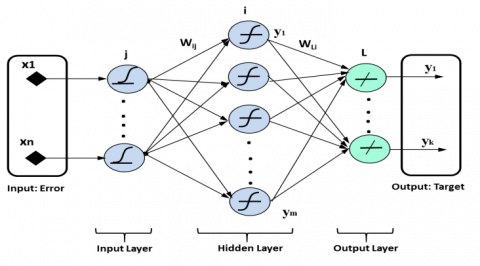Figure 4. Structure of a standard neural network

By taking all these concepts in consideration, it can be noted that it is especially the experiment, and the number of tests, which help one to search the number of neurons and more exactly an optimal architecture for a given problem.

The general feed forward structure shown in Figure 4, consists of three layers, input-output, and hidden layers with n, k, and m neurons, respectively.

The signal propagation of each layer is described in detail as follows:

${{u}_{j}}=X(j)\left( j=1,2,\cdots n \right)$  (24)

where, uj denotes the jth neuron used for the input layer, and the n neurons number depend on the process complexity.

The input and output on the hidden layer are:

${{v}_{i}}=\underset{j=1}{\overset{n}{\mathop{\sum }}}\,{{\omega }_{ij}}{{u}_{j}}$  (25)

${{y}_{i}}=f({{v}_{i}})=f(\underset{j=1}{\overset{n}{\mathop{\sum }}}\,{{\omega }_{ij}}{{u}_{j}})\left( i=1,2,\cdots m \right)$  (26)

where, yi is the total input of ith neuron used for the hidden layer, wijis the weight values, and f (*) denotes the activation function.

## 5.png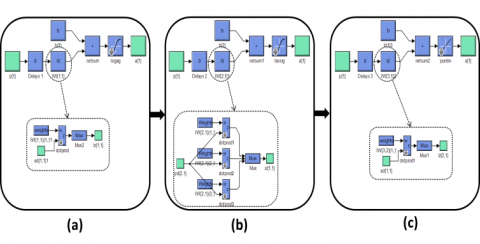Figure 5. Block diagram of numerical controller: (a) Input Layer; (b) Hidden Layer; (c) Output Layer

The input of the output layer is:

${{z}_{L}}=\underset{L=1}{\overset{k}{\mathop{\sum }}}\,{{\omega }_{Li}}{{y}_{i}}=\underset{L=1}{\overset{k}{\mathop{\sum }}}\,{{\omega }_{Li}}f({{v}_{i}})$  (27)

The total output of the neural network can be represented by the following equation:

\begin{align} & {{y}_{k}}=f({{z}_{L}})=f(\underset{L=1}{\overset{k}{\mathop{\sum }}}\,{{\omega }_{Li}}f({{v}_{i}})) \\ & =f(\underset{L=1}{\overset{k}{\mathop{\sum }}}\,{{\omega }_{Li}}f(\underset{j=1}{\overset{n}{\mathop{\sum }}}\,{{\omega }_{ij}}{{u}_{j}})) \\\end{align}   (28)

Based on the self-structure, the optimal architecture of Multilayer Perceptron (MLP) takes, in this case, a single neuron in the input-output layers, and only one hidden layer of 3 neurons for designing the numerical controllers (Figure 5), which replace the four PI current-controllers and the PI MPPT-controller (Figure 6), in order to maintain a high dynamic performance even when detuning occurs. At first, we are training the MLP to the PI controller by presenting 6000 samples to the network with a maximal error of 10-20, the number of epochs count maximum 103 with an iteration step of five. Then, the MLP must be trained in order to adjust and to find the adequate weights, which adapts the input (error) with the output (target). The backpropagation algorithm named Levenberg–Marquardt (LM) is used to train the networks. The neural network gives almost the same output pattern for the same or nearby values of input.

Each neuron in this architecture has a corresponding threshold b, as shown in Figure 5.

The adaptation of the weights and thresholds values can represent by the following equations:

${{\xi }_{i}}(k+1)={{\xi }_{i}}(k)+\Delta {{\xi }_{i}}$  (29)

$\Delta {{\xi }_{i}}=-\eta \frac{\partial \varepsilon (k)}{\partial {{\xi }_{i}}(k)}$  (30)

with: ξi = wi or bi.

$\varepsilon (k)=\frac{1}{2}\underset{i=1}{\overset{m}{\mathop{\sum }}}\,{{e}_{i}}^{2}(k)$  (31)

${{e}_{i}}(k)={{y}_{i\_desird}}-{{y}_{i\_actually}}$  (32)

where, ɛ(k) is the instantaneous sum of squared output errors SSE, e(k) is the error, η is a learning rate that takes it as a constant value between 0 and 1. The initial values of the weights and thresholds are chosen randomly, and the final values after the training process are presented in the following sets Z, H, and M.

The activation functions of the input and the hidden layers are respectively the "logsig" "tansig" function, and the "linear" function is used for the output layer. Its learning is carried out using the error-based gradient propagation algorithm.

This method, which is an approximation of Newton’s method, has been shown to be one of the fastest algorithms for training moderate size MLPs and ensures the best convergence towards a minimum of the quadratic error.

The general parameters (number of layers, max error, activation functions) can be successfully applied in other applications, as shown in literatures [31, 32]. However, the proper design parameters (number of neurons, weights values, threshold values), can be applied with success only in the same applications (NMPPT-NFOC-DSIG).

• Set Z: for MPPT controller

ω1= [6.9e+04]; b1= [-3.32e+04]

ω2= [1.1e+05; 7.5e+04;-1.2e+05]; b2=[-9.7e3;-2.9e4;6.6e3]

ω3= [3.6e+06, 3.6e+06, -1.8e+06]; b3= [1.8e+06]

• Set H: for Id controller

ω1= [-5.58e-05]; b1= [-0.67]

ω2= [-1.6e+02; 2.1e+02; 1.7e+02]; b2=[53.3;-73.9;-56.9]

ω3= [3.1e+03, -1.9e+03, -2.3e+03]; b3= [1.1e+03]

• Set M: for Iq controller

ω1= [-29.19]; b1= [16.31]

ω2= [2.8e+02; -1.9e+02; 67.4]; b2= [-61.6;35.3;1.1e2]

ω3= [-8 e+02, 1.5e+02, 2e+06]; b3= [-1.9e+06]

7. Grid Side Power Control

The main objective of the grid side converter control is to inject into the grid the optimal power provided by the wind turbine. The grid active and reactive powers are controlled by decoupled voltage oriented control, through the regulation of d-q axis grid currents (Figure 6) . In order to have the grid current vector in phase with the grid voltage vector, the reference reactive power Q should be zero.

The DC-Bus voltage is expressed by:

${{V}_{dc}}=\frac{1}{c}\int{{{i}_{c}}dt}$  (33)

where:

${{i}_{c}}={{i}_{dc}}-{{i}_{ond}}$

To ensure the constant rated value of the DC-Bus voltage, a PI-controller was used, in the regulation loop, in order to generate the reference capacitive current Idc.

$i_{dc}^{*}=PI(u_{dc}^{*}-{{u}_{dc}})$  (34)

The reference grid active power is presented by:

${{P}^{*}}={{V}_{dc}}({{i}_{m}}-i_{dc}^{*})={{P}_{dc\_m}}-P_{dc}^{*}$  (35)

The d-q reference currents obtained in the grid are expressed by:

$\left[ \begin{matrix} i_{dg}^{*} \\ i_{qg}^{*} \\\end{matrix} \right]=\frac{1}{v_{dg\_mes}^{2}-v_{qg\_mes}^{2}}\left[ \begin{matrix} {{P}^{*}} & {{Q}^{*}} \\ -{{Q}^{*}} & {{P}^{*}} \\\end{matrix} \right]\left[ \begin{matrix} {{v}_{dg\_mes}} \\ {{v}_{qg\_mes}} \\\end{matrix} \right]$  (36)

The reference voltages are given by:

\left\{ \begin{align} & v_{md}^{*}=v_{dg}^{*}+{{v}_{dg\_mes}}-w{}_{s}{{L}_{f}}{{i}_{qg\_mes}} \\ & v_{mq}^{*}=v_{qg}^{*}+{{v}_{qg\_mes}}-w{}_{s}{{L}_{f}}{{i}_{dg\_mes}} \\\end{align} \right.  (37)

\left\{ \begin{align} & v_{dg}^{*}=PI(i_{dg}^{*}-{{i}_{dg\_mes}}) \\ & v_{qg}^{*}=PI(i_{qg}^{*}-{{i}_{qg\_mes}}) \\\end{align} \right.   (38)

## 6.png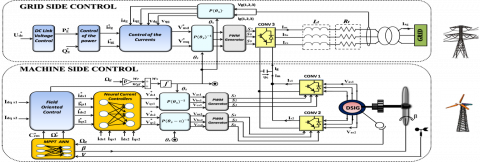Figure 6. Block diagram of the global grid-connected wind turbine based on the DSIG

8. Simulation Results and Discussion

The simulation results of the control system presented in this paper, were obtained by using Matlab /Simulink software for the wind speed profile indicated in Figure 7. The simulated model was implemented under discrete time mode and for a sampling time Ts=10 µs. The rated power of the DSIG is 1.5 MW, with parameters shown in the appendix.

## 7.png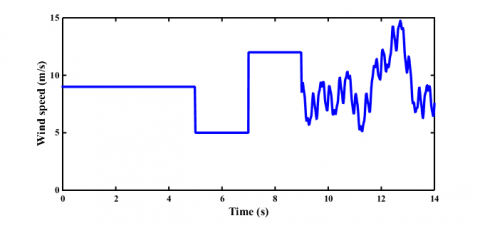Figure 7. Profile of wind speed

## 8a.png(a)

## 8b.png(b)

## 8c.png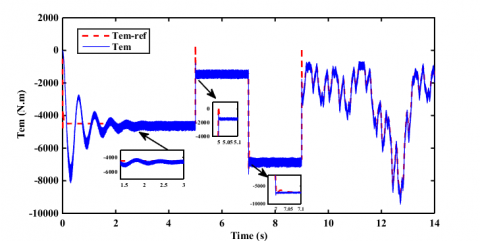(c)

## 8d.png(d)

## 8e.png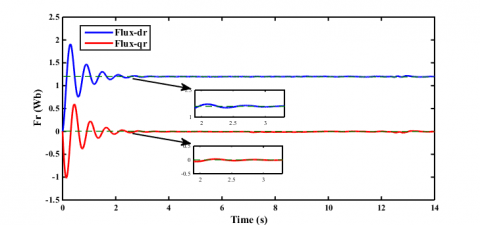(e)

Figure 8. Simulation results using ANN controllers: (a) generator speed (b) active power (c) electromagnetic torque (d) stator current of star 1 and star 2 (e) rotor flux

The results in Figure 8 are related to the ANN controller, whereas the results of Figure 9 are for the PI controller. Both control schemes are tested under the same conditions and for different wind speeds.

The first test shown in Figure 8 and Figure 9 is a comparison between the PI controllers and the ANN controllers, in terms of response speed, transient oscillations, and tracking of imposed trajectory.

## 9a.png(a)

## 9b.png(b)

## 9c.png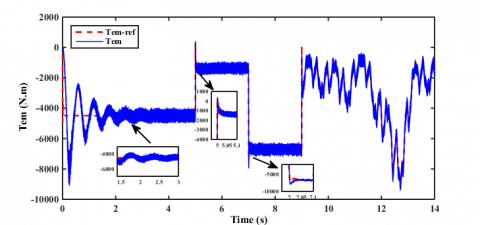(c)

## 9d.png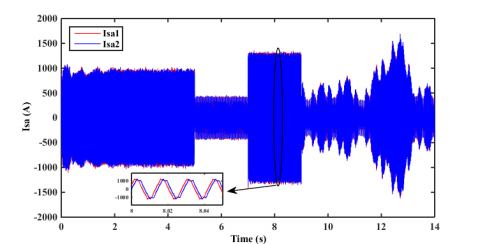(d)

## 9e.png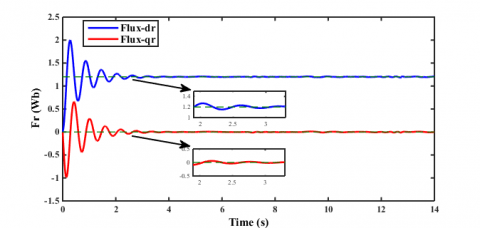(e)

Figure 9. Simulation results using PI controllers: (a) generator speed (b) active power (c) electromagnetic torque (d) stator current of star 1 and star 2 (e) rotor flux

It is clear from the results of rotor speed, active power and electromagnetic torque (Figures 8.a.b.c and Figures 9.a.b.c) that the ANN controller has a fast response speed, as compared to the PI controller. It can be also seen that both controllers have a good tracking trajectory; however, the ANN controller has better performance especially under transient oscillations, which can eliminate overshoot regardless of speed changes. On the other side the PI controller generates an overshoot at the times of speed variation, as shown at the instants t = 5 s and t = 7 s.

In terms of static error, the ANN controller presents lower values than the PI controller, especially at high speed, as shown in Figure 8.b and Figure 9.b, between t=7 s and t= 9 s.

Figure 8.d and Figure 9.d show the evolution of the DSIG stator currents for the first phase of each star. The currents are sinusoidal, and shifted by 30°. The direct and quadratic rotor fluxes of the DSIG are illustrated in Figure 8.e and Figure 9.e. They are decoupled, where the component of the direct rotor flux follows its reference, and the component of the quadratic rotor flux is null.

## 10.png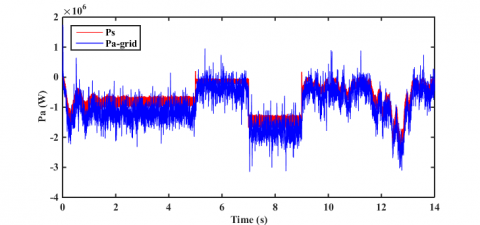Figure 10. Grid active power

## 11.pngFigure 11. Grid reactive power

From Figures 10 and 11, it can be observed that the grid active and reactive powers smoothly follow their references, under all the considered operating conditions.

In Figure 12 the DC bus voltage tracks its reference with good stability and accuracy, showing this way the control robustness against wind variations. Figure 13 shows that the unity power factor is always achieved.

## 12.png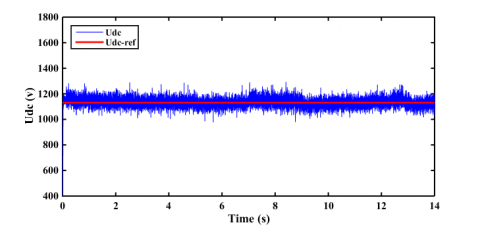## 13.png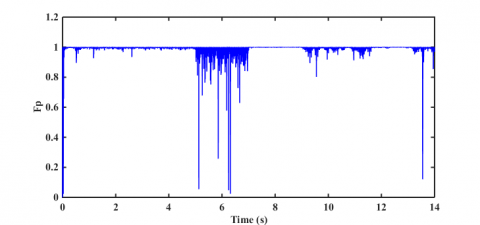Figure 13. Power factor in grid side

9. Comparative Studies Between PI and ANN Controllers

In order to show the feasibility of the proposed control when using the PI and neural network controllers, a system with and without parametric variations is considered.

Figures 14-15 and Table 1 are specified for PI and ANN controllers, to compare both performances under the same conditions.

## 14.pngFigure 14. Generator speed comparison using PI and neural controllers with an increased moment of inertia (J +80%)

## 15a.png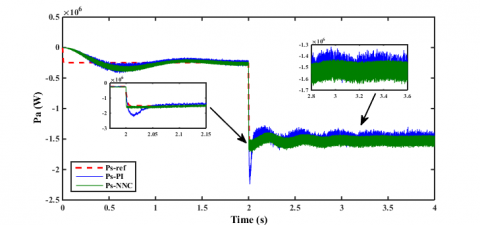(a)

## 15b.png(b)

Figure 15 Active power simulation results verifying the controllers robustness (a) variation of Ls value by 50%; (b) variation of Lr value by 50% and Ls value by 50% at t= 3s

Table 1. Controllers performance analysis

 Performance Normal conditions Abnormal conditions PI ANN PI ANN Response time at low speed (s) 1.7 1.5 1.7 1.5 Response time at high speed (ms) 2.5 2 3 2 Overshoot (%) 10 0 40 2.5 Static error in the speed (rad/s) 1.5 0.02 4 0.5 Static error in the active power (%) 2.5 0.05 0.25 0 Tracking and Robustness Good Excellent Bad Good THD Is (%) 4.60 1.46 8.23 1.62

It can be seen that the FOC based on ANN controllers shows better control performance of the machine-side converter, as compared to the PI controller based on FOC, showing this way the effectiveness of the proposed control scheme under normal and abnormal conditions.

10. Conclusion

This paper has been dedicated to the modeling and synthesis of the control laws of a grid-connected WECS based on the DSIG machine. An NMPPT-NFOC scheme is proposed for a machine-side converter control in order to have good tracking of the reference values. The proposed controller avoids the sensitivity of the conventional PI controllers based-MPPT-FOC against the parametric and wind speed variations. On the other side, a VOC based on the PI controllers is used to control the DC-link voltage and the grid active and reactive powers. Simulation results show that as opposed to the classical control, the proposed method has a smooth and close tracking of the reference values with insensitivity to parameter variations, which confirm that the considered method is an effective solution for DSIG drives.

Future work will focus on the application of the proposed ANN based-control to the grid-side converter, since the control stability against the filter parameter variations and grid normal and abnormal conditions is one of the research interests of the authors.

Acknowledgment

This work was supported by the European Regional Development Fund (ERDF) through the Operational Programme for Competitiveness and Internationalization (COMPETE 2020), under Project POCI-01-0145-FEDER-029494, by National Funds through the FCT - Portuguese Foundation for Science and Technology, under Projects PTDC/EEI-EEE/29494/2017, UIDB/04131/2020, and UIDP/EEA/04131/2020.

Nomenclature
 V Wind speed (m/s) Cp Power coefficient -- R Radius of the blade (m) ρ Air density -- λ Speed ratio -- β Pitch angle (deg) Lm Magnetizing inductance (H) J Inertia moment (Kgm2) G Gear box gain -- Lr , Ls Rotor and Stator inductance (H) Rr , Rs Rotor and Stator resistance (Ohm) ωs Synchronous reference Speed ωr Rotor electrical angular speed Vds Vqs d-q stator voltages (V) Vdg Vqg d-q grid voltages (V) Vdc DC-bus voltage (V) Ødr Øqr d-q rotor flux (Wb) Ids Iqs d-q stator currents (A) Idg Iqg d-q grid currents (A) Idc DC-bus current (A) Ps Active Power (W) Qs Reactive Power (Var) Tem Electromagnetic Torque (N.m) wi Neuron weight -- bi Neuron threshold --
Appendix
 Radius of the turbine R=35,25m Optimal relative speed λopt = 8,1 Maximum power coefficient Cpmax = 0,48 Gear box gain G= 90 DSIG rated power Pr = 1,5 MW RMS voltage value V= 400 V Number of pole pairs p= 2 Stator resistance Rs1=Rs2=0,008 Ohm Stator inductance Ls1=Ls2=0,134 mH Magnetizing inductance Lm=0,0045 H Rotor resistance Rr=0,007 Ohm Rotor inductance Lr=0,067 mH Inertia J=100 Kg.m2 Viscous Coefficient fv =2,5 Nm s/rd Filter Inductance Lf=0,001 H Filter resistance Rf=0,001 ohm Capacitance of the DC-Bus C=0,072 F
References

 Bu, F., Hu, Y., Huang, W., Zhuang, S., Shi, K. (2014). Wide-speed-range-operation dual stator-winding induction generator DC generating system for wind power applications. IEEE Transactions on Power Electronics, 30(2): 561-573. https://doi.org/10.1109/TPEL.2014.2308222

 Rajendran, S., Jena, D. (2015). Backstepping sliding mode control of a variable speed wind turbine for power optimization. Journal of Modern Power Systems and Clean Energy, 3(3): 402-410. https://doi.org/10.1007/s40565-015-0106-2

 Kouzi, K., Nait-Said, M.S., Hilairet, M., Berthelot, É. (2008). A fuzzy sliding-mode adaptive speed observer for vector control of an induction motor. In IEEE International Conference on Industrial Electronics IECON.

 Achouri, F., Mendil, B. (2019). Wind speed forecasting techniques for maximum power point tracking control in variable speed wind turbine generator. International Journal of Modelling and Simulation, 39(4): 246-255. https://doi.org/10.1080/02286203.2019.1572691

 Kashkooli, M.A., Madani, S.M., Lipo, T.A. (2019). Improved direct torque control for a DFIG under symmetrical voltage dip with transient flux damping. IEEE Transactions on Industrial Electronics, 67(1): 28-37. https://doi.org/10.1109/TIE.2019.2893856

 Yaichi, I., Semmah, A., Wira, P. (2019). Direct power control of a wind turbine based on doubly fed induction generator. European Journal of Electrical Engineering, 21(5): 457-464. https://doi.org/10.18280/ejee.210508

 Zouggar, E.O., Chaouch, S., Abdeslam, D.O., Abdelhamid, A.L. (2019). Sliding control with fuzzy type-2 controller of wind energy system based on doubly fed induction generator. Instrumentation Mesure Metrologie, 18(2): 137-146. https://doi.org/10.18280/i2m.180207

 Qais, M.H., Hasanien, H.M., Alghuwainem, S. (2018). A grey wolf optimizer for optimum parameters of multiple PI controllers of a grid-connected PMSG driven by variable speed wind turbine. IEEE Access, 6: 44120-44128. https://doi.org/10.1109/ACCESS.2018.2864303

 Yang, L., Huang, T.M., Deng, L., Zeng, Y.F., Huang, S.D. (2019). Analysis on chaotic mechanism of direct-drive permanent magnet synchronous generators based on Lyapunov stability theory. European Journal of Electrical Engineering, 21(6): 531-537. https://doi.org/10.18280/ejee.210607

 Benakcha, M., Benalia, L., Ammar, A., Bourek, A. (2019). Wind energy conversion system based on dual stator induction generator controlled by nonlinear backstepping and pi controllers. International Journal of System Assurance Engineering and Management, 10(4): 499-509. https://doi.org/10.1007/s13198-018-0734-9

 Chekkal, S., Lahaçani, N.A., Aouzellag, D., Ghedamsi, K. (2014). Fuzzy logic control strategy of wind generator based on the dual-stator induction generator. International Journal of Electrical Power & Energy Systems, 59: 166-175. https://doi.org/10.1016/j.ijepes.2014.02.005

 Singh, K., Kumar Singh, G. (2015). Modeling and analysis of six-phase self-excited induction generator using mixed stator current and magnetizing flux as state-space variables. Electric Power Components and Systems, 43(20): 2288-2296. https://doi.org/10.1080/15325008.2015.1082162

 Benakcha, M., Benalia, L., Tourqui, D.E., Benakcha, A. (2018). Backstepping control of dual stator induction generator used in wind energy conversion system. International Journal of Renewable Energy Research, 8(1): 384-395. https://doi.org/10.30521/jes.351269

 Amimeur, H., Aouzellag, D., Abdessemed, R., Ghedamsi, K. (2012). Sliding mode control of a dual-stator induction generator for wind energy conversion systems. International Journal of Electrical Power & Energy Systems, 42(1): 60-70. https://doi.org/10.1016/j.ijepes.2012.03.024

 Marouani, K., Nounou, K., Benbouzid, M., Tabbache, B., Alloui, H. (2015). DC bus voltage control of a power generation system based on a dual star induction generator. International Review of Electrical Engineering (IREE), 11(6): 686. https://doi.org/10.15866/iree.v11i6.9769

 Mata, J., de Miguel, I., Duran, R.J., Merayo, N., Singh, S.K., Jukan, A., Chamania, M. (2018). Artificial intelligence (AI) methods in optical networks: A comprehensive survey. Optical Switching and Networking, 28: 43-57. https://doi.org/10.1016/j.osn.2017.12.006

 Shah, B., Trivedi, B.H. (2012). Artificial neural network based intrusion detection system: A survey. International Journal of Computer Applications, 39(6): 13-18. https://doi.org/10.5120/4823-7074

 Ameur, F., Kouzi, K. (2014). Optimized PI and fuzzy speed vector control of dual stator induction generator used in a variable speed wind. International Journal of Electrical Energy, 2(1): 74-81. https://doi.org/10.12720/ijoee.2.1.74-81

 Yaichi, I., Semmah, A., Wira, P. (2020). Control of doubly fed induction generator with maximum power point tracking for variable speed wind energy conversion systems. Periodica Polytechnica Electrical Engineering and Computer Science, 64(1): 87-96. https://doi.org/10.3311/PPee.14166

 Tahir, K., Belfedal, C., Allaoui, T., Doumi, M. (2015). Proposal of a new hybrid control strategy for dynamic performance improvement of wound field synchronous generator-based wind turbines. Journal of Renewable and Sustainable Energy, 7(4): 043113. https://doi.org/10.1063/1.4926768

 Aouzellag, D., Ghedamsi, K., Berkouk, E.M. (2009). Network power flux control of a wind generator. Renewable Energy, 34(3): 615-622. https://doi.org/10.1016/j.renene.2008.05.049

 Djeriri, Y. (2020). Lyapunov-based robust power controllers for a doubly fed induction generator. Iranian Journal of Electrical and Electronic Engineering, 16(4): 551-558. https://doi.org/10.22068/IJEEE.16.4.551

 Kaid, I., Guemana, M., Hafaifa, A., Kouzou, A. (2018). Identification and modeling of wind turbine variables for efficient energetic conversion. In 2018 15th International Multi-Conference on Systems, Signals & Devices (SSD), pp. 1293-1297. https://doi.org/10.1109/SSD.2018.8570520

 Ihedrane, Y., El Bekkali, C., Bossoufi, B. (2017). Direct and indirect field oriented control of DFIG-generators for wind turbines variable-speed. In 2017 14th International Multi-Conference on Systems, Signals & Devices (SSD), pp. 27-32. https://doi.org/10.1109/SSD.2017.8166915

 Wu, B., Lang, Y., Zargari, N., Kouro, S. (2011). Fundamentals of wind evergy conversion system control. In Power Conversion and Control of Wind Energy Systems. https://doi.org/10.1002/9781118029008.ch2

 Chatterjee, S., Chatterjee, S. (2018). A novel speed sensor-less vector control of Dual Stator Induction machine with space vector based advanced 9-zone hybrid PWM for grid connected wind energy generation system. Electric Power Systems Research, 163: 174-195. https://doi.org/10.1016/j.epsr.2018.02.021

 Bojoi, R., Lazzari, M., Profumo, F., Tenconi, A. (2002). Digital field oriented control for dual three-phase induction motor drives. IEEE Transactions on Industry Applications, 39(3): 752-760. https://doi.org/10.1109/TIA.2003.811790

 Bojoi, R., Levi, E., Farina, F., Tenconi, A., Profumo, F. (2006). Dual three-phase induction motor drive with digital current control in the stationary reference frame. IEE Proceedings-Electric Power Applications, 153(1): 129-139. https://doi.org/ 10.1049/ip-epa:20050215

 Chekkal, S., Aouzellag, D., Ghedamsi, K., Amimeur, H. (2011). New control strategy of wind generator based on the dual-stator induction generator. In 2011 10th International Conference on Environment and Electrical Engineering, 1-4. https://doi.org/10.1109/EEEIC.2011.5874593

 Tir, Z., Soufi, Y., Hashemnia, M.N., Malik, O.P., Marouani, K. (2017). Fuzzy logic field oriented control of double star induction motor drive. Electrical Engineering, 99(2): 495-503. https://doi.org/10.1007/s00202-016-0377-2

 Massoum, A., Meroufel, A., Djeriri, Y. (2014). Artificial neural network based direct torque control of doubly fed induction generator. Journal of Electrical Engineering, 14(2): 8-8.

 Mesai Ahmed, H., Djeriri, Y., Bentaallah, A. (2018). Robust power control of DFIG using artificial neural networks for a wind energy conversion system based energy storage unit. 2nd International Symposium on Mechatronics & Renewable Energy ISMRE'2018 El-Oued, December.

 Yaramasu, V., Wu, B., Sen, P.C., Kouro, S., Narimani, M. (2015). High-power wind energy conversion systems: State-of-the-art and emerging technologies. Proceedings of the IEEE, 103(5): 740-788. https://doi.org/10.1109/JPROC.2014.2378692